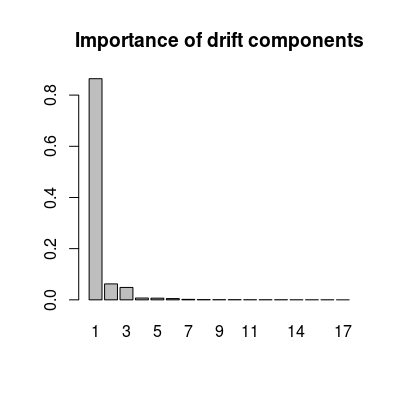Dataset UNIMANdnoise.

Description

The dataset contains statistics on a multi-variate drift sub-space for the long-term UNMIAN dataset of 1000 samples over 8 classes.

Details

The subspace was evaluated via common principal component analysis (power algorithm). Only three classes (analytes at maximum concentration) are used in the computation for more accurate estimation. The importance of the drift components is computed as a projected variance of sensor array data of the given three classes onto the components.

The datasets contains four variables:

 dspace A square 17 x 17 matrix of the drift subspace. Columns are drift component vectors. ndvar A vector of length 17 with the importance of the components. centered Boolean indicating whether the UNIMAN data were centered before the computation. scaled Boolean indicating whether the UNIMAN data were scaled before the computation.

Examples

data(UNIMANdnoise) str(UNIMANdnoise)
List of 4 \$ centered: logi TRUE \$ dspace : num [1:17, 1:17] 0.274 0.312 0.28 0.278 0.278 ... \$ ndvar : num [1:17] 0.86425 0.06216 0.04846 0.00693 0.00647 ... \$ scaled : logi TRUE
 17 17
# importance of 17 drift components head(UNIMANdnoise\$ndvar)
 0.864246632 0.062158036 0.048459565 0.006925524 0.006465561 0.005053250
barplot(UNIMANdnoise\$ndvar, names.arg=1:length(UNIMANdnoise\$ndvar), main="Importance of drift components")